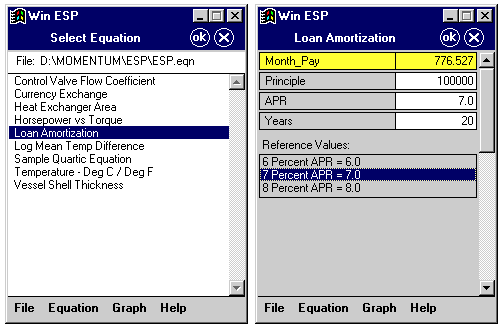Software tour of PocketESP pocket solver is coming soon.   In the meantime, download a free working demo copy to see ESP for yourself.

PocketESP (Pocket Equation Solver Program) allows you to enter equations and solve for any variable in the equation.   The applications are virtually unlimited; business, engineering, finance, medicine, etc..  Variable values are persistent, that is the values are preserved from one equation to another.  This allows equations to be linked into sets to solve complex problems.   For instance, if the user built a set of financial equations and a variable named "payment" changed in one equation, the new value would also be updated in any other equation containing the variable "payment".   Each equation can contain up to 16 variables and all standard functions are supported, i.e. log, ln, cos, acos, cosh, exp.  Equations can have reference values which are named numeric constants and are displayed when the equation is displayed.   ESP can store any number of equations in a file and you can have any number of files, (limited only by memory).    The package contains two programs: "PocketESP" for your pocket PC (Win CE), and "WinESP" which runs on your desktop PC (Win 95/98/NT), and the equation files work with both programs. (Price \$49)